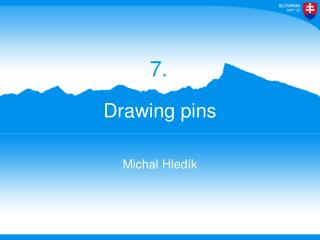DownloadDownload PresentationDrawing pins

# Drawing pins

Download Presentation## Drawing pins

- - - - - - - - - - - - - - - - - - - - - - - - - - - E N D - - - - - - - - - - - - - - - - - - - - - - - - - - -
##### Presentation Transcript

1. 7. Drawing pins Michal Hledík

2. 7. Drawing pins A drawing pin (thumbtack) floating on the surface of water near another floating object is subject to an attractive force. Investigate and explain the phenomenon.Is it possible to achieve a repulsive force by a similar mechanism?

3. Video of attracting • +picture of pins

4. Content • Deformation of water surface • Attraction of pins • Mechanism • Calculating the motion • Theory vs. experiments • Repulsion of objects • Mechanism • Attracting/repelling boundary

5. 1. Deformationof water surface

6. Forces analysis Gravity Buoyant force Surface tension Force equilibrium

7. Force equilibrium Eq. (1) Only unknown quantities

8. Water displacement Absent water – compensated by surface tension Pressure – hydrostatic

9. Water displacement Resulting function: ` ` ` ` Dominic Vella,L. Mahadevan,The ‘‘Cheerios effect,’’(2005)

10. Finding contact angle Eq. (1): Predicted angle:

11. Contact angle measurement Distant light source Analyzing size of shade of the pin Pin on water

12. Measuring the contact angle Applying Snell’s law, fitting contact angle (size of the shade) Contact angle:

13. Shape of water surface [mm] [mm]

14. 2. Attraction of pins

15. Why are they attracting? 2 pins on water – inclined to each other Mass of a pin > mass of water displaced Potential energy of water and pin – decreases as pin descends

16. Determining the acceleration Horizontal motion: β

17. Slope of one pin Given by the deformation of water surface by the other pin Our approximation:

18. Drag force Assuming …position Video analysis and fit

19. Fitting the drag coefficient Distance passedx [m] Time[s]

20. Acceleration  distance in time Dependence of acceleration on distance and velocity Numerical solution

21. Attracting – experiment

22. Theory vs. experiment Distance of the pins [mm] Time[s]

23. 3. Repelling objects

24. Repulsive force Object wetted by water  acts downwards  object floats up

25. Repelling objects Plastic caps from pins – float upwards Behavior depends on weight There is a critical mass – does not repel or attract

26. Both caps wetted by water

27. + A little weight on the yellow cap

28. Empty cap and a cap with a weight Distance between the caps [cm] 0,204g 0,162g Greater mass  stronger repulsion 0,062g 0,041g 0,027g Time[s]

29. Conclusion We explained the mechanism of • floating, attraction, repulsion Determined the deformation of water surface Described the motion quantitatively • theory correlates with experiments Found the border between attraction/repulsion Thank you for your attention

30. Appendices 7. Drawing pins

31. Drawing pin “dipole” – attracts different objects on different sides

32. Water displacement • Boundary conditions: • Solution: ` Dominic Vella,L. Mahadevan,The ‘‘Cheerios effect,’’(2005)

33. Critical mass • Water is not deformed • Surface tension resultant force = 0

34. Critical mass • Pin caps – bent edges • Mass theoretically: • Depth: • Mass experimentally: